• 通过模型比较,优选并组合了Sigmoid函数的偏最小二乘、线性的支持向量回归、径向基的支持向量回归和Sigmoid函数的支持向量回归4个单模型,集成算法优化后发现,梯度提升树算法的预测结果最优。与单模型的预测结果相比,...
• # -*- coding: utf-8 -*- """ Created on Tue May 15 17:23:42 2018 @author: eagle ""...# =========================================...# 线性回归预测波士顿房价 # ====================...
# -*- coding: utf-8 -*-
"""
Created on Tue May 15 17:23:42 2018

@author: eagle
"""
# =============================================================================
# 线性回归预测波士顿房价
# =============================================================================

from sklearn.datasets import load_boston
from sklearn.cross_validation import train_test_split
import numpy as np
from sklearn.preprocessing import StandardScaler

from sklearn.linear_model import LinearRegression  #线性回归
from sklearn.linear_model import SGDRegressor   #快速随机梯队下降(Stochastic Gradient Descend)

from sklearn.metrics import r2_score,mean_squared_error,mean_absolute_error

#加载房价数据
boston = load_boston()      #共506条数据
print(boston.DESCR)
X = boston.data
y = boston.target

#训练数据和测试数据分割
X_train,X_test,y_train,y_test = train_test_split(X,y,test_size = 0.25,random_state = 33)
print('The max target value is',np.max(boston.target)) #最大值
print('The min target value is',np.min(boston.target)) #最小值
print('The average target value is',np.mean(boston.target)) #平均值

#标准化
ss_X = StandardScaler()
ss_y = StandardScaler()

X_train = ss_X.fit_transform(X_train)
X_test = ss_X.transform(X_test)
y_train = ss_y.fit_transform(y_train.reshape(-1,1))
y_test = ss_y.transform(y_test.reshape(-1,1))

#用线性回归模型中的LinearRegression和SGDRegression进行预测
lr=LinearRegression()
lr.fit(X_train,y_train)
lr_y_predict = lr.predict(X_test)
#SGDRegression
sgdr=SGDRegressor()
sgdr.fit(X_train,y_train)
sgdr_y_predict = sgdr.predict(X_test)

#评价
print('The scroe of LinearRegression is:',lr.score(X_test,y_test))
print('The r2-score of LinearRegression is:',r2_score(y_test,lr_y_predict))
print('The mean_squared_error of LinearRegression is',mean_squared_error(ss_y.inverse_transform(y_test),ss_y.inverse_transform(lr_y_predict)))
print('The mean_absolute_error of LinearRegression is',mean_absolute_error(ss_y.inverse_transform(y_test),ss_y.inverse_transform(lr_y_predict)))

#评价
print('The scroe of SGDRegression is:',sgdr.score(X_test,y_test))
print('The r2-score of SGDRegression is:',r2_score(y_test,sgdr_y_predict))
print('The mean_squared_error of SGDRegression is',mean_squared_error(ss_y.inverse_transform(y_test),ss_y.inverse_transform(sgdr_y_predict)))
print('The mean_absolute_error of SGDRegression is',mean_absolute_error(ss_y.inverse_transform(y_test),ss_y.inverse_transform(sgdr_y_predict)))
代码如上，与书中不同的是，需要进行数组的变换，否则会报错。y_train = ss_y.fit_transform(y_train.reshape(-1,1))
y_test = ss_y.transform(y_test.reshape(-1,1))运行结果表明：使用随机梯度下降方法估计参数的性能不及解析方法的线性回归，但是在数据规模庞大的情况下，随机梯队法会非常高效。（Scikit-learn官网建议数据规模超过10万推荐使用随机梯度法参数估计模型）
展开全文• 另外，因为这是个预测准确值的任务，所以它也是个回归任务。 注： 1）监督学习（Supervised learning）：根据已有的数据集，知道输入和输出结果之间的关系。根据这种已知 的关系，训练得到一个最优的模型。也就是...
目 录

1 需求分析

2 数据探索

3 数据预处理

4 构建模型与评估

1 需求分析

这是个输出模型，模型的输出是某个地区的房价。显而易见是监督学习的任务。另外，因为这是个预测准确值的任务，所以它也是个回归任务。

1）监督学习（Supervised learning）：根据已有的数据集，知道输入和输出结果之间的关系。根据这种已知的关系，训练得到一个最优的模型。也就是说，在监督学习中训练数据既有特征(feature)又有标签(label)，通过训练，让机器可以自己找到特征和标签之间的联系，在面对只有特征没有标签的数据时，可以判断出标签。通俗一点，可以把机器学习理解为我们教机器如何做事情。回归(Regression）、分类（Classification)

2）无监督学习(Unsupervised Learning )，我们不知道数据集中数据、特征之间的关系，而是要根据聚类或一定的模型得到数据之间的关系。可以这么说，比起监督学习，无监督学习更像是自学，让机器学会自己做事情，是没有标签（label）的。

2 数据探索

2.1 初步探索

1) 导入数据

import pandas as pd

def LoadData(file):
return pd.read_csv('D:/Project/Anaconda/Lib/site-packages/sklearn/datasets/data/'+file,encoding='gbk')
data = LoadData('California.csv')
data.head(5)

查看导入的结果，每个数据有十个特征，分别是经度，纬度，房屋年龄中位数，房间总数，卧室总数，地区人口数，家庭数量，收入中位数，房价中位数以及一个位置属性（是否接近湾区）。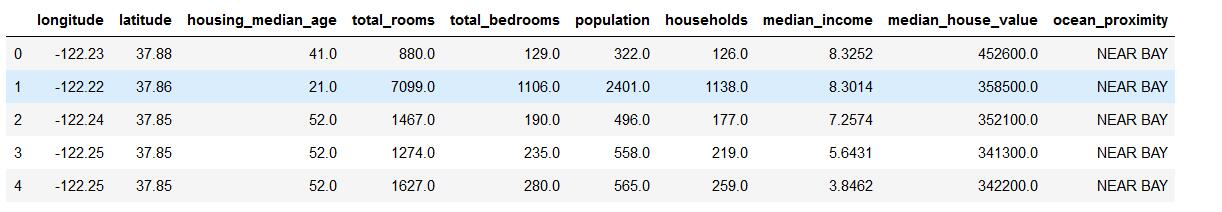2) 使用Info（）和describe()函数查看数据属性

data.info()

info()函数
功能： 给出样本数据的相关信息概览 ：行数，列数，列索引，列非空值个数，列类型，内存占用
使用格式：DataFrame.info(verbose=None, memory_usage=True, null_counts=True)
参数解释：

verbose：True or False，字面意思是冗长的，也就说如何DataFrame有很多列，是否显示所有列的信息；如果为否，那么会省略一部分；
memory_usage：True or False，默认为True，是否查看DataFrame的内存使用情况；
null_counts：True or False，默认为True，是否统计NaN值的个数

运行结果如图：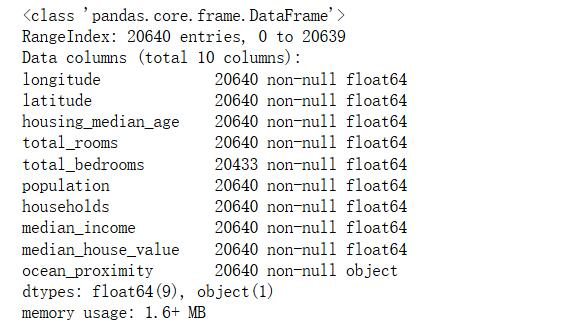可以看到有的属性有缺失值，有的属性类型不为数值型。

data.describe()

功能：直接给出样本数据的一些基本的统计量，包括均值，标准差，最大值，最小值，分位数等。
使用格式：data.describe()
可以传递一些参数，如percentiles = [0.2,0.4,0.6,0.8]就是指定0.2，0.4，0.6，0.8分位数，而不是默认的0.25，0.5，0.75分位数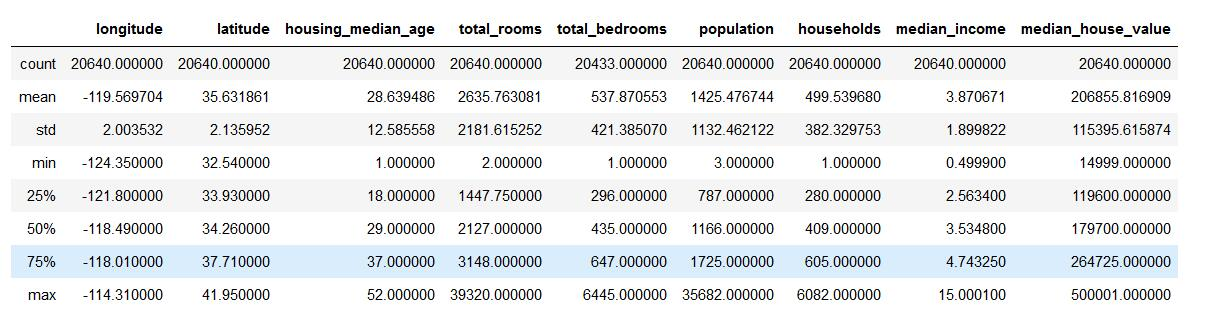拿房屋年龄中位数举例，它的25%显示是18，表示有25%的地区房子年龄少于18年，50%的地区房子年龄少于29年，75%的房子年龄少于37年。这三个属性有助于我们了解数据的基本分布情况。

2.2 数据集分割

from sklearn.model_selection import train_test_split

house_train,house_test = train_test_split(data, test_size=0.2, random_state=24)
print("train :",len(house_train)," test:",len(house_test) )

train : 16512  test: 4128

功能是从样本中随机的按比例选取traindata和testdata，形式为：
X_train,X_test, y_train, y_test = cross_validation.train_test_split(train_data,train_target,test_size=0.4, random_state=0)

参数解释：
train_data：所要划分的样本特征集
train_target：所要划分的样本结果
test_size：样本占比，如果是整数的话就是样本的数量
random_state：是随机数的种子。
随机数种子：其实就是该组随机数的编号，在需要重复试验的时候，保证得到一组一样的随机数。
比如你每次都填1，其他参数一样的情况下你得到的随机数组是一样的。但填0或不填，每次都会不一样。
随机数的产生取决于种子，随机数和种子之间的关系遵从以下两个规则：
种子不同，产生不同的随机数；种子相同，即使实例不同也产生相同的随机数。

2.3 相关性探索

housing = house_train.copy()
corr_matrix = housing.corr()
corr_matrix["median_house_value"].sort_values(ascending=False)

运行结果如下，这是计算每个属性之间的标准相关系数（也称作皮尔逊相关系数）,查看每个属性和median_house_value的相关系数，数值在[-1,1]之间。 接近1时，说明两个特征有很明显的正相关关系;相反则是有很明显的负相关关系;如果是0，则说明没有相关关系。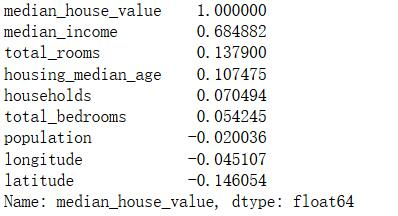再看一下热力图：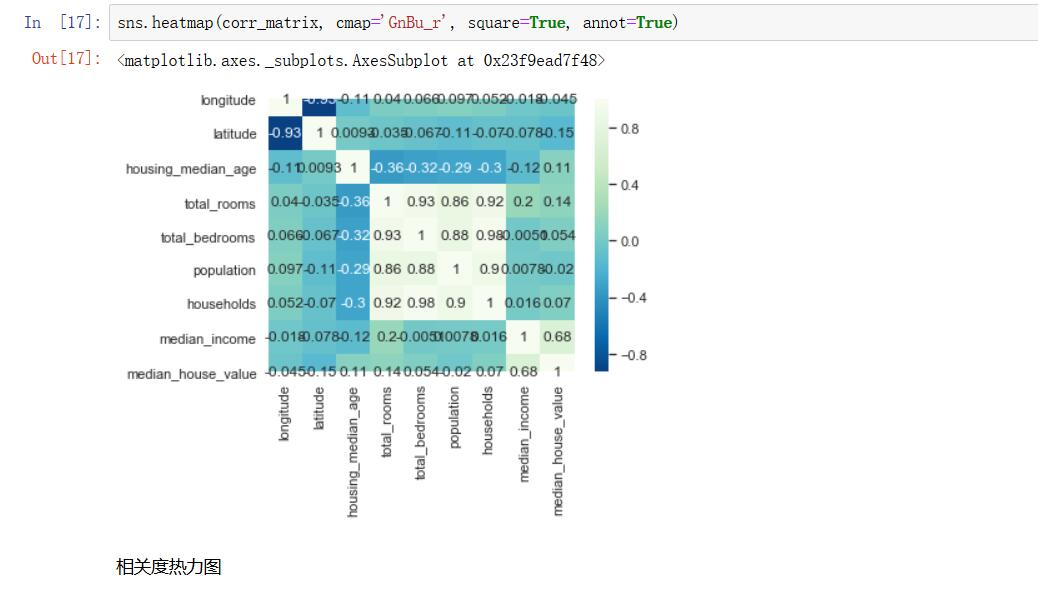heatmap热度图,拿到一批数据一般会求特征之间的相关系数，可以用padas直接求出来相关系数，放到heatmap，可以很清楚的看到两个特征的相关程度，这是一个固定的数据思维。

3 数据预处理

数据预处理是构建特征工程的基本环境，并且是提高算法精确度的有效手段。数据预处理需要根据数据本身的特性进行，不同的格式有不同的处理要求，比如无效的要剔除，缺失值要回填和冗余维度的选择等。

3.1 SKLearn进行数据预处理介绍

1）无量纲化

在机器学习算法实践中，我们往往有着将不同规格的数据转换到同一规格，或不同分布的数据转换到某个特定分布的需求，这种需求统称为将数据无量纲化。数据的无量纲化可以是线性的，也可以是非线性的。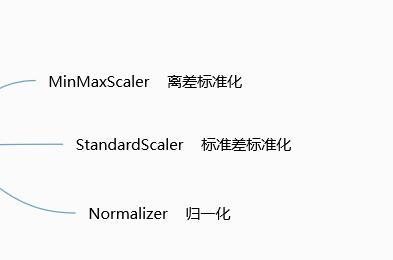2）缺失值处理

数据处理过程中，不可避免的会出现很多空值，这时就需要我们通过业务经验选择性的进行数值填充，尽量保证模型的准确性。

sklearn.impute.SimpleImputer(missing_values=nan,strategy=‘mean’, fill_value=None, verbose=0, copy=True)

参数介绍：
1.missing_values:告诉SimpleImputer，数据中的缺失值长什么样，默认空值np.nan
2.strategy:我们填补缺失值的策略，默认均值。
输入“mean”使用均值填补（仅对数值型特征可用）
输入“median"用中值填补（仅对数值型特征可用）
输入"most_frequent”用众数填补（对数值型和字符型特征都可用）
输入“constant"表示请参考参数“fill_value"中的值（对数值型和字符型特征都可用）
3.fill_value:当参数startegy为”constant"的时候可用，可输入字符串或数字表示要填充的值，常用0。
4.copy:默认为True，将创建特征矩阵的副本，反之则会将缺失值填补到原本的特征矩阵中去。

3）分类型特征处理

由于很多的机器学习算法是计算数值型数据的，但现实情况下特征数据中包含很多文本数据，又不能忽略掉。在这种情况下，为了让数据适应算法和库，我们必须将数据进行编码，即是说，将文字型数据转换为数值型。

preprocessing.LabelEncoder
标签专用，能够将分类转换为分类数值，是对不连续的数字或者文本进行
编号（连续的会是同一个编号）。
preprocessing.OrdinalEncoder
特征专用，能够将分类特征转换为分类数值，其主要处理有序变量。
preprocessing.OneHotEncoder
独热编码，创建哑变量，其处理名义变量
举个例子：

自然状态码为：000,001,010,011,100,101
独热编码为：000001,000010,000100,001000,010000,100000
可以这样理解，对于每一个特征，如果它有m个可能值，那么经过独热编码后，就变成了m个二元特征
（如成绩这个特征有好，中，差变成one-hot就是011, 010, 001）。并且，这些特征互斥，每次只有一个激活；而且，可以表示原始数据的大小。）

4）连续型特征处理

sklearn.preprocessing.Binarizer类
根据阈值将数据二值化（将特征值设置为0或1），用于处理连续型变量。

preprocessing.KBinsDiscretizer
这是将连续型变量划分为分类变量的类，能够将连续型变量排序后按顺序分箱后编码。

3.2 本项目中的实现

from  sklearn.pipeline  import Pipeline
from sklearn.impute import SimpleImputer
from sklearn.preprocessing import StandardScaler
from sklearn.compose import ColumnTransformer
from sklearn.preprocessing import OneHotEncoder

#拆分数据,获得特征矩阵H以及只含有median_house_value作为真实的label值，y值
housing=house_train.drop("median_house_value",axis=1)
housing_labels=house_train["median_house_value"].copy()
housing_copy = housing.drop("ocean_proximity",axis=1)#去掉干扰值

num_attribs = list(housing_copy)
cat_attribs = ['ocean_proximity']

num_type_pipeline=Pipeline([
('simputer',SimpleImputer(strategy="median")),     #用中位数填充
('std_scaler',StandardScaler())

])

full_pipeline = ColumnTransformer([
("num", num_type_pipeline, num_attribs),
("cat", OneHotEncoder(), cat_attribs),        #使用独热编码
])

housing_prepared = full_pipeline.fit_transform(housing)#x值
housing_prepared.shape

输出：(16512, 13)

介绍这个流水线式的处理思想：

在Sklearn当中有三大模型：Transformer 转换器、Estimator 估计器、Pipeline 管道

Transformer转换器 (StandardScaler，MinMaxScaler)。
Estimator 估计器（LinearRegression、LogisticRegression、LASSO、Ridge），所有的机器学习算法模型，都被称为估计器。
Pipeline 管道：将Transformer、Estimator 组合起来成为一个大模型。输入→□→□→□→■→ 输出                                     □：Transformer ； ■：Estimator ；Transformer放在管道前几个模型中，而Estimator 只能放到管道的最后一个模型中。
pipeline用于对特征处理、数据转换、回归或分类等多个步骤进行串联，功能是实现多个estimator的串行处理。

featureunion用于对特征的处理，功能是实现多个transformer的并行处理，最终输出它们的结果的并集。每个transformer的输入都是全部的原始特征。

ColumnTransformer用于对特征的处理，功能是针对不同的列做不同的处理，最终输出各自结果的合集。与featureunion不同的是，它各个transformer的输入是原始特征的一部分。

4 构建模型与评估

根据分析过程中输出和输入的关系，又可以分类成回归分析、聚类分析、分类和关联规则分析等。
根据输入的特征，可以分为监督学习、无监督学习和半监督学习等。
from sklearn.ensemble import GradientBoostingRegressor
from sklearn.metrics import mean_squared_error,explained_variance_score,mean_absolute_error,median_absolute_error,r2_score

#预测模型
GBR_house = GradientBoostingRegressor().fit(housing_prepared,housing_labels)
GBR_housing_predictions = GBR_house.predict(housing_prepared)

#评价模型
GBR_mse = mean_squared_error(housing_labels,GBR_housing_predictions)
GBR_ab = mean_absolute_error(housing_labels,GBR_housing_predictions)
GBR_va = explained_variance_score(housing_labels,GBR_housing_predictions)
GBR_mab = median_absolute_error(housing_labels,GBR_housing_predictions)
GBR_r2 = r2_score(housing_labels,GBR_housing_predictions)
GBR_rmse = np.sqrt(GBR_mse)

#输出评价结果
print('GBR回归模型的平均值绝对值为：',GBR_ab)
print('GBR回归模型的中值绝对值为：',GBR_mab)
print('GBR回归模型的可解释方差值为：',GBR_va)
print('GBR回归的决定系数为：',GBR_r2)
print ('GBR回归模型的标准差为：',GBR_rmse)

评价结果输出：

GBR回归模型的平均值绝对值为： 37309.044287613804
GBR回归模型的中值绝对值为： 26495.989202691184
GBR回归模型的可解释方差值为： 0.7861164571729515
GBR回归的决定系数为： 0.7861164571729515
GBR回归模型的标准差为： 53398.51873035388

参考链接：sklearn.ensemble.GradientBoostingRegressor官方文档：https://scikit-learn.org/stable/modules/generated/sklearn.ensemble.GradientBoostingRegressor.html#sklearn.ensemble.GradientBoostingRegressor

GBR模型解释：https://blog.csdn.net/xlsj228/article/details/105122816

https://blog.csdn.net/zhsworld/article/details/102951061

评估指标解释：
mean_squared_error：均方差（Mean squared error, MSE），
该指标计算的是拟合数据和原始数据对应样本点的误差的平方和的均值，其值越小说明拟合效果越好。

mean_absolute_error：平均绝对误差（Mean Absolute Error, MAE），
用于评估预测结果和真实数据集的接近程度的程度，其值越小说明拟合效果越好。

explained_variance_score：解释回归模型的方差得分，其值取值范围是[0,1]，
越接近于1说明自变量越能解释因变量的方差变化，值越小则说明效果越差。

r2_score：判定系数，其含义是也是解释回归模型的方差得分，其值取值范围是[0,1]，
越接近于1说明自变量越能解释因变量的方差变化，值越小则说明效果越差。

展开全文大数据 python 机器学习
• 这是近期在kaggle上的一场回归预测的竞赛，官方所给的数据集有1491个样本，79个特征，需要我们进行一定的特征处理然后选取适合的模型来进行预测。本文采取两种先进的回归技术进行预测，分别是随机森林和梯度提升树...
这是近期在kaggle上的一场回归预测的竞赛，官方所给的数据集有1491个样本，79个特征，需要我们进行一定的特征处理然后选取适合的模型来进行预测。本文采取两种先进的回归技术进行预测，分别是随机森林和梯度提升树以及它俩的集成。
题目 https://www.kaggle.com/c/house-prices-advanced-regression-techniques

数据处理

由于其特征较多，首先进行特征筛选，筛选规则是所有的Str列，如果某一个属性占了95%以上或者某一列有一半以上的是NaN值，则把这些列删掉，剩余的Str列进行0，1，2，3……编码，然后填补缺失值为0。数据处理代码如下：

houseprice_data = pd.read_csv("./data/train.csv")#读取数据
datalength = len(houseprice_data)#数据量

#数据处理：

houseprice_col = list(houseprice_data.columns)#获取列名
print(houseprice_data.columns)
delcol_list = []#要删除的列名列表

for i,col in enumerate(houseprice_col):
if type(houseprice_data[col]) == str:#如果是字符串列
if np.array(houseprice_data[col].value_counts()).max()>datalength*0.95:#如果次数最多的大于90%
delcol_list.append(col)#填入要删除的列名之中

for col in list(houseprice_data.columns):#删掉nan为一半的列
if houseprice_data[col].count() < datalength/2:
delcol_list.append(col)
delcol_list.append('Id')#把id加进去
delcol_list.append('FireplaceQu')#特殊列加进去
houseprice_data_one = houseprice_data.drop(delcol_list,axis=1)#drop掉delcollist

for j,col in enumerate(list(houseprice_data_one.columns)):#遍历新列
if type(houseprice_data[col]) == str:
values = pd.Categorical(houseprice_data_one[col]).codes
houseprice_data_one[col] = values#对字符串列进行编码

houseprice_data_one = houseprice_data_one.fillna(0)#填缺失值为0

随机森林

处理完数据之后，我们先用随机森林进行预测：

#进行训练预测

X = np.array(houseprice_data_one.drop('SalePrice',axis=1))
Y = np.array(houseprice_data_one['SalePrice'])

x_train,x_test,y_train,y_test = train_test_split(X,Y,test_size=0.25,random_state=1)#切分数据集

clf = RandomForestRegressor()#随机森林
clf.fit(x_train,y_train)
print("拟合率：",clf.score(x_train,y_train))
print("预测率：",clf.score(x_test,y_test))

#拟合率： 0.9673565610627388
#预测率： 0.9035892586277517

交叉验证与归一化

我们只切分了一次数据集，这只是在一部分数据上的结果，不足以证明模型的有效性，接下来我们做交叉验证，并对X进行归一化处理：

#交叉验证
kf1 = KFold(n_splits=10,shuffle=False)#10折交叉验证
#随机森林
clfre = RandomForestRegressor(n_estimators=210,max_depth=30,max_features=30)

scaler = MinMaxScaler()#归一化处理
X_scaler = scaler.fit_transform(X)

#交叉验证得分
for retrain,retest in kf1.split(X_scaler,Y):
clfre.fit(X_scaler[retrain],Y[retrain])
retrain_scores.append(clfre.score(X_scaler[retrain],Y[retrain]))
retest_scores.append((clfre.score(X_scaler[retest],Y[retest])))

#得分情况
print("交叉验证训练分数：",np.array(retrain_scores).mean())
print("交叉验证测试分数：",np.array(retest_scores).mean())

#交叉验证训练分数： 0.9818125682413038
#交叉验证测试分数： 0.87427725293159

交叉验证之后所能达到的最高分数在0.874左右，在kaggle平台测试分数要比不进行交叉验证要高，说明我们的模型泛化能力增强了。

梯度提升树

接下来看梯度提升树：

train_scoresx = []
test_scoresx = []

kfx = KFold(n_splits=10,shuffle=False)#10折交叉验证
clfx = GradientBoostingRegressor(n_estimators=3000, learning_rate=0.05, max_depth=3, max_features='sqrt', loss='huber', random_state=42)#梯度提升树

scalerx = MinMaxScaler()#归一化处理
X_scalerx = scalerx.fit_transform(X)

for train,test in kfx.split(X_scalerx,Y):
clfx.fit(X_scalerx[train],Y[train])
train_scoresx.append(clfx.score(X_scalerx[train],Y[train]))
test_scoresx.append((clfx.score(X_scalerx[test],Y[test])))

#得分情况
print("交叉验证训练分数：",np.array(train_scoresx).mean())
print("交叉验证测试分数：",np.array(test_scoresx).mean())

#交叉验证训练分数： 0.994345303778351
#交叉验证测试分数： 0.8911478084451863

模型集成

梯度提升树交叉验证得分要比随机森林高的多，可以达到0.891。接下来我们将两个模型进行集成：

#均方误差函数：
def score(y_test,y_true):
return (1 - ((y_test - y_true)**2).sum() / ((y_true - y_true.mean())**2).sum())

#梯度提升树与随机森林集成
test_scores = []

kfx = KFold(n_splits=10,shuffle=False)#10折交叉验证

clfx = GradientBoostingRegressor(n_estimators=3000, learning_rate=0.05, max_depth=3, max_features='sqrt', loss='huber', random_state=42)#梯度提升树
clfr = RandomForestRegressor(n_estimators=210,max_depth=30,max_features=30)#随机森林

scalerx = MinMaxScaler()#归一化处理
X_scalerx = scalerx.fit_transform(X)

for train,test in kfx.split(X_scalerx,Y):
clfx.fit(X_scalerx[train],Y[train])
clfr.fit(X_scalerx[train],Y[train])
score((clfx.predict(X_scalerx[test])+clfr.predict(X_scalerx[test]))/2,Y[test])#模型预测结果平均值集成方法

#得分情况
print("集成分数：",np.array(r2score).mean())

#集成分数：0.889


集成分数要比梯度提升树第一点，但是在kaggle平台得分比梯度提升树要高，所有集成的模型适应性更强。如果要评价这三个模型，应该用专用的模型评价指标评价，可以利用roc_auc_score来评价。

数据获取：
链接：https://pan.baidu.com/s/16Ej8Q5I8ep1g8QBuStN8dw 密码：ix62
展开全文随机森林
• 利用日PM2.5数据分别KNN、逻辑回归、随机森林、梯度提升机进行了比较研究 源代码分享在了github上：https://github.com/yopo520/PM2.5-prediction-of-Baoji
利用日PM2.5数据分别使用KNN、逻辑回归、随机森林、梯度提升机进行了比较研究

源代码分享在了github上：https://github.com/yopo520/PM2.5-prediction-of-Baoji

展开全文机器学习 python
• EL之Boosting之GB(DTR)：利用梯度提升法解决回归(对多变量的数据集+实数值评分预测)问题 目录 输出结果 设计思路 核心代码 输出结果 设计思路 核心代码 xList = [] labels = [] ...
• 使用线性回归，具有L2正则化的线性回归，具有L1正则化的线性回归，随机森林回归梯度提升算法，具有深度限制的决策树回归，无深度限制的决策树回归，主成分分析，PCA之后的线性回归的房价预测，没有最大深度限制的...
• 使用梯度下降的方法以后速度和预测效果都得到了提升。 本来想在最后加上对预测结果的可视化显示，可惜并没有成功。数据可视化方面还是需要再多学习一下。 import matplotlib.pyplot as plt import numpy as np
• 梯度提升树模型在智慧交通预测的运用，朱宏岩，胡燕祝，目前对于道路交通拥堵的预测多采用单一预测模型，例如线性回归方法与时间序列预测方法，这些预测方法维度较为单一，尽管在一些简数据挖掘
• 今天学习了梯度提升决策树（Gradient Boosting Decision Tree, GBDT），准备写点东西作为记录...梯度提升算法的思想是通过调整模型，让损失函数的值不断减小， 然后将各个模型加起来作为最终的预测模型。而梯度提升...
• 梯度提升是一种用于回归和分类问题的机器学习技术，其产生的预测模型是弱预测模型的集成，如采用典型的决策树作为弱预测模型，这时则得到了所谓的梯度提升树（GBDT）。GBDT是在传统机器学习算法里面是对真实分布拟合...机器学习 集成学习
• GBDT（Gradient boosting ...生成梯度提升树的案例： 具体步骤： （1）根据原始数据生成回归树 （2）然后计算残差，用残差替代y，生成新的数据。 残差=真实值-预测值 （3）用新生成的数据生成第2颗生成树 （...
• 梯度提升（Gradient ...梯度提升（梯度增强）是一种用于回归和分类问题的机器学习技术，其产生的预测模型是弱预测模型的集成，如采用典型的决策树 作为弱预测模型，这时则为梯度提升树（GBT或GBDT）。像其他提升...机器学习 GBDT
• 接着上篇博客聊到的回归树，我们说到要预测一个班级的同学的成绩，用平均数数不妨为一个还不错的值。我们假设这个班级除了他自己，还有3位同学(请忽略为什么这个班级人这么少。。。)的成绩为50,60,70 ...
• 梯度提升是一种用于回归和分类问题的机器学习技术，其产生的预测模型是弱预测模型的集成，如采用典型的决策树作为弱预测模型，这时则得到了所谓的梯度提升树（GBDT）。GBDT是在传统机器学习算法里面是对真实分布拟合...
• 【翻译自： Histogram-Based Gradient ...鉴于它在实践中在各种数据集上表现出色，它可能是针对结构化（表格）分类和回归预测建模问题的最受欢迎的技术之一。梯度提升的主要问题是训练模型的速度较慢。在具有成千...
• 梯度提升是一种用于回归和分类问题的机器学习技术，其产生的预测模型是弱预测模型的集成，如采用典型的决策树作为弱预测模型，这时则得到了所谓的梯度提升树（GBDT）。GBDT是在传统机器学习算法里面是对真实分布拟合...GBDT 机器学习 集成学习
• 提升树：提升方法采用加法模型（基函数的线性组合）与前向分布算法，以决策树为基函数的提升方法为...梯度提升树：而对于其他损失函数，提出了利用负梯度表示残差的近似值。 为什么采用损失函数的负梯度？ L(y,f(...
• 0、GBDT利用的是CART回归树损失函数的一阶负梯度来近似模拟当前模型的真实值（实际上是上一轮模型预测值与真实值的残差），从而达 到减小损失函数的目的。 1、GBDT基模型必须是CART回归树（无论是分类问题还是...机器学习
• 梯度提升是决策树算法的集合。鉴于它在实践中在各种数据集上表现出色，它可能是针对结构化（表格）分类和回归预测建模问题的最受欢迎的技术之一。梯度提升的主要问题是训练模型的速度较慢。在具有成千...算法 python 机器学习 人工智能 深度学习
• GBDT梯度提升树原理剖析在前面的文章中，我写了ID3及CART决策树的思路，但我们只是将其用来做分类。注意到CART的名字：Classification And Regression Tree，当我们利用其进行回归时，它的名字就变成了回归树。GBDT...算法 GBDT python
• 上篇博客已经讲了梯度提升树，但只讲了回归，本节讲一下分类，和一些进阶方法。 GBDT 分类 其实 GBDT 分类和回归思路基本相同，只是由于分类的标签是离散值，无法拟合误差， 解决办法有两种：一种是用指数损失...
• Boosting算法是一种把若干个分类器整合为一个分类器的方法，也就是一种集成分类方法（Ensemble Method）...从数值的角度来看，优化是用梯度下降来解决的（这就是为什么这种技术也被称为梯度提升）。 同样，最佳值..R语言 Adaboost 动态可视化
• 算法目标：图中的x，y图表示的就是，曲线上的点就是这个回归树所预测到的残差的预测值，我们最后要的就是预测值接近真实的残差就是去拟合这个残差（Fitted residual），让损失函数（Loss）最小。 例子 初始化的10个...算法 机器学习
• 梯度提升回归树算法，更改公式、加注释使其更加容易理解  增加章节2.GBDT优缺点，6.梯度提升分类树算法 1.GBDT概念 以决策树为基学习器的集成学习算法具有预测精度高的优势，树的集成算法主要有随机森林和GBDT。...GBDT 提升树...